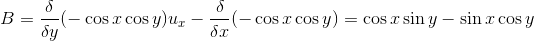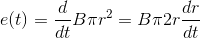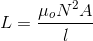# MCQs on Electromagnetic Fields

##### Page 14 of 20. Go to page 1 2 3 4 5 6 7 8 9 10 11 12 13 14 15 16 17 18 19 20
01․ Find H for centre of solenoid having finite length d = 1 unit where N = no of turns = 500, I = 2 A.
100.
500.
1000.
None of these.

From Biot Savarts law, magnetic field intensity for a solenoid is given as H = NI/ux.

02․ A magnetic vector potential is given by the expression A = (- cos x) (cos y) uz. The flux density at the origin is
0.
– uy.
– 2 ux.
2 ux.

B = curl of ASince flux density at origin (x = 0, y = 0) so B = 0.

03․ A circular loop has its radius increasing at a rate of 2 m/s. The loop placed perpendicular to a constant magnetic field of 0.5 wb/m2. When the radius of the loop is 4 m. the emf induced in the will be
0.8 π V.
0.4 π V.
8 π V.
Zero.

Area of the loop A = π r2 Magnetic of unit emf induced dQ/dt.04․ A magnetic field B = 5 × 10-2 uz T exerts a force on 0.02 m conductor along the x-axis. The conductor carried a current of 5 mA in the – ux direction. What force must be applied to the conductor to hold it in position
5 × 10-5 uy N.
- 5 × 10-5 uy N.
5 × 10-5 uz N.
5 × 10-5 ux N.

F = IL × B Applied forced Fa = - (IL × B) Since Fa force hold conductor position. So the force will be opposed.

05․ The hysteresis loop of a magnetic material has an area of 5 cm2 with the scale given as 1 cm = 2 AT and 1 cm = 50 mwb At 50 Hz; the total hysteresis loss is
15 W.
20 W.
25 W.
50 W.

Power loss in watt given as, Ph = W h v.f Where Wh = energy density loss V = Volume of material In this case, Wh.V = area of hysteresis loop = 5 cm2.

06․ A wire 1 m long carries a current of 5A and is at an angle of 30o with B = 1.5 wb/m2. Magnitude of force is
2 N.
2.5 N.
3.75 N.
5 N.

F = BIL sinθ.

07․ A negative point charge q = - 40 nC is moving with velocity of 6 × 106 m/s in a direction specited by unit vector uy + 0.6 uz. Find the magnitude of force vector on the moving particle by the field B = 2 ux + 3 uy + 5 uz mT and E = 2 ux - 3 uy + 5 uz KV/m
144 μN.
244 μN.
1440 μN.
1144 μN.

Apply F = Q(E + V × B).

08․ The Kirchhoff’s current law is implict in expression
Divergence of D = ρv.
Divergence of B = 0.
Surface integral of (j.ds) = 0.
Curl of H = J + (δ D)/(δ t).

Current at a node is zero, current entering = current leaving. So, surface integral of (j.ds) = 0.

09․ The magnetic field at any point on the axis of current carrying circular coil will be
parallel to axis.
perpendicular to axis.
at an angle 45o with the axis.
zero.

With coil x – y plane, the field will be in uz direction.

10․ A coil of 300 turns is wound on a non magnetic core having a mean circumference of 300 mm and a cross sectional area of 300 mm2. The inductance of the coil corresponding to a magnetizing current of 6 A will be
1.1304 μH.
3.768 μH.
37.68 μH.
113.04 μH.

Inductance of coil,<<<1213141516>>>